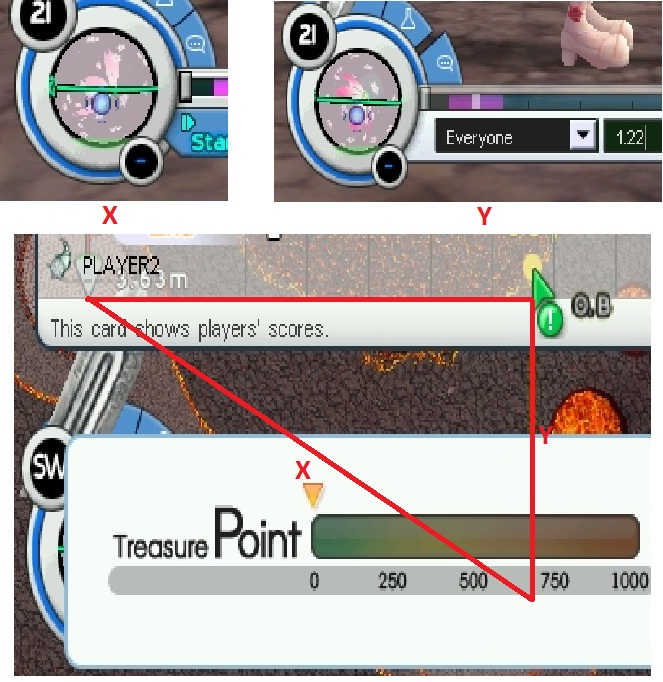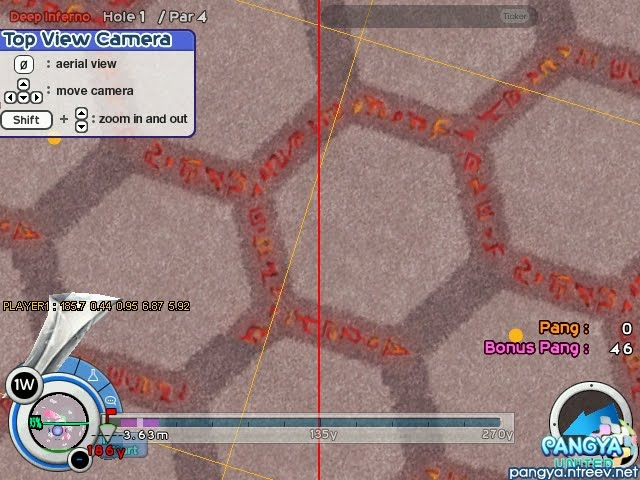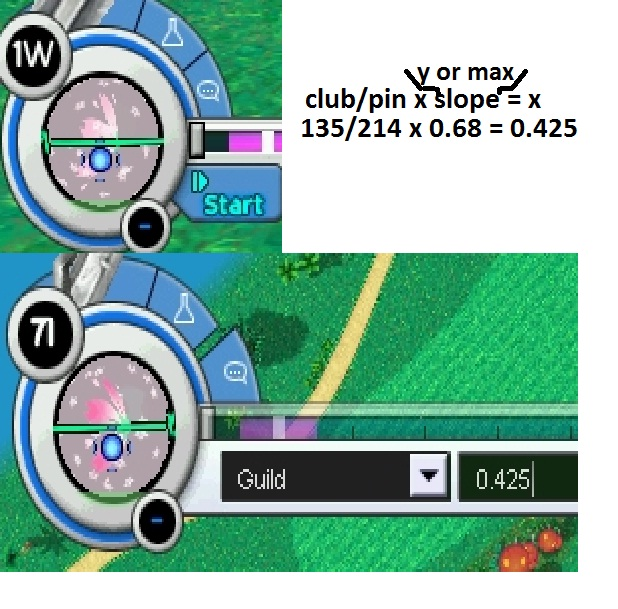Pangya Slope Reading Guide by meinards

Few people have asked me to make a guide which would explain how some top players in the world read slopes. Since I worked on this with some help from top TH players(who learned this method from JP players, lol, linking for the win) credit goes to them. This is my interpretation of the method, so some details might not be 100% accurate.

The first difference in this method is that you will no longer count breaks. As some of you might have already noticed, slopes might look the same but have different effects on the shot. What this means is that there are more breaks which you can’t see.

How to measure slope?This tool shows how I measure the slope. Entire line is divided in 9 equal breaks. Each | is representing 0.1 in length. I start measuring from the very edge of the circle(note that this is not the only way!).

So, how to apply that to slope reading? Trigonometry, of course! This screenshot below shows the basic idea behind it.X – club distance, Y – pin distance.

Basically, you have a lot of ways to do this. What I prefer is using simple functions: x/y = (slope for x)/(slope for y) — Very simple.

Another popular method is using difference in angles so you don’t have to figure out how long is the club distance(and sometimes it’s a must, for example when club has X instead of O at the end. If it has X, the distance is NOT what you see on the screen!).

Now, how this works when calculating your shot?

If you look at the triangle image, you will see number 1.22 on top right. That’s the slope length for Y which also is the maximum slope you can get on that spot(note that it will be pair number of breaks at all times!). Basically you end up spinning around while looking for it.

~67.5y/186=0.36
0.36 x 1.22=0.44

Now to modify this coefficient for my power-cut. This is straight forward since it’s cut % x 0.44. For the situation I had it was 234.0 cut or 86.67%.

0.44 x 0.867 = 0.38

Finally I multiply it by my final HWI(not aim!).

2.5 x 0.38 = 0.95So, how exactly do I measure the slope?(credits to Shunya/Anaume http://penguinshunya.blog.so-net.ne.jp/ )

You simply add a, b, c and d together(for exact length look at the very first image) and multiply by 2(note that this is how I do it, it might differ from person to person. For example, I’d start with D and move to A which is backwards from what Shunya/Anaume does).

Some examples:Again, credits to Shunya/Anaume(JP/US) for sharing the information with the world, Himari/Gigglegimmi(TH) for a lot of translation and basic concepts and Serie(JP) from whom I first saw this method put in use.

P.S. I’m willing to answer questions about slopes you might stumble upon, so if you’re interested, take screenshots!

P.S.S. Check out some Shunya’s videos! http://zoome.jp/penguinshunya/diary/13

Or some of my ”full” games on youtube such as –